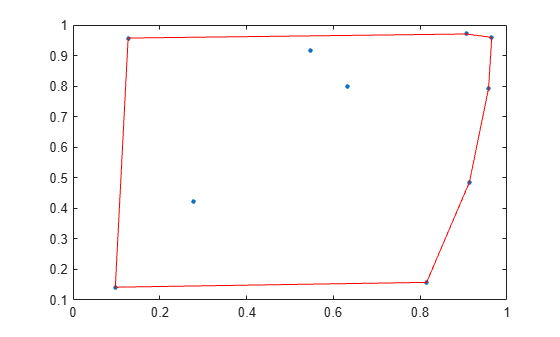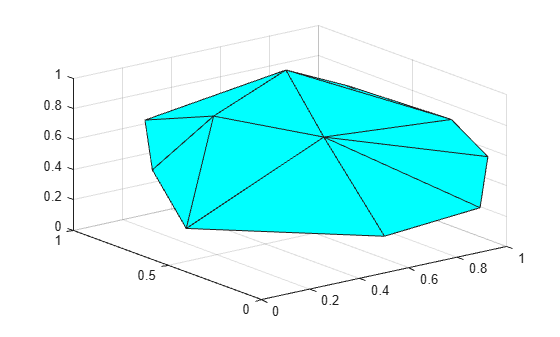# convexHull

Convex hull of Delaunay triangulation

## Syntax

``C = convexHull(DT)``
``````[C,v] = convexHull(DT)``````

## Description

example

````C = convexHull(DT)` returns the vertices of the convex hull of a Delaunay triangulation.```

example

``````[C,v] = convexHull(DT)``` also returns the area or volume bounded by the convex hull.```

## Examples

collapse all

Compute and plot the convex hull of a 2-D Delaunay triangulation.

Create a Delaunay triangulation from a set of 2-D points.

```x = gallery('uniformdata',[10,1],0); y = gallery('uniformdata',[10,1],1); DT = delaunayTriangulation(x,y);```

Compute the convex hull.

`C = convexHull(DT);`

Plot the triangulation and highlight the convex hull in red.

```plot(DT.Points(:,1),DT.Points(:,2),'.','MarkerSize',10) hold on plot(DT.Points(C,1),DT.Points(C,2),'r') ```Compute and plot the convex hull of a 3-D Delaunay Triangulation.

Create a Delaunay triangulation from a 3-D set of points.

```P = gallery('uniformdata',[25,3],1); DT = delaunayTriangulation(P);```

Compute the convex hull and the volume bounded by the convex hull.

`[C,v] = convexHull(DT);`

Display the volume and plot the convex hull.

`v`
```v = 0.3561 ```
```trisurf(C,DT.Points(:,1),DT.Points(:,2),DT.Points(:,3), ... 'FaceColor','cyan')```## Input Arguments

collapse all

Delaunay triangulation, specified as a scalar `delaunayTriangulation` object.

Data Types: `delaunayTriangulation`

## Output Arguments

collapse all

Convex hull vertices, returned as a column vector or matrix of vertex IDs.

• When `DT` is a 2-D triangulation, `C` is a column vector containing the sequence of vertex IDs around the convex hull. The vertex IDs are the row numbers of the vertices in the `Points` property.

• When `DT` is 3-D triangulation, `C` is a 3-column matrix containing the connectivity list of triangle vertices in the convex hull.

Data Types: `double`

Area or volume of the convex hull, returned as a scalar.

Data Types: `double`